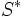# Involution fusion pattern

This article defines an equivalence relation over the collection of groups. View a complete list of equivalence relations on groups.
This term is related to: Classification of finite simple groups
View other terms related to Classification of finite simple groups | View facts related to Classification of finite simple groups

## Contents

This article is about a definition in group theory that is standard among the group theory community (or sub-community that dabbles in such things) but is not very basic or common for people outside.
Two groups$G$ and$G^*$ are said to have the same involution fusion pattern if there is an isomorphism$x \mapsto x^*$ from a 2-Sylow subgroup$S$ of$G$ to a 2-Sylow subgroup$S^*$ of$G^*$ such that$x,y \in S$ are conjugate in$G$ if and only if$x^*, y^*$ are conjugate elements in$S^*$.